Anzeige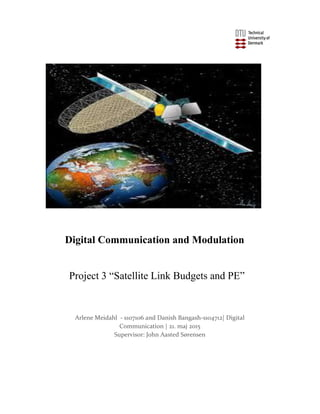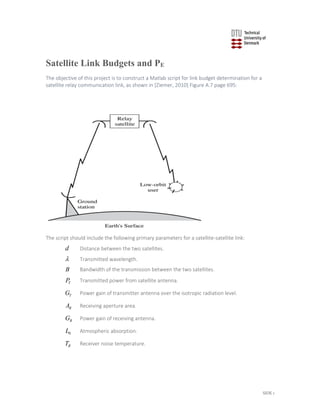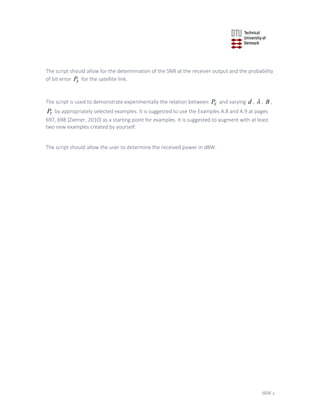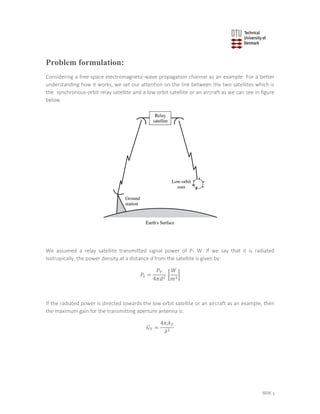Anzeige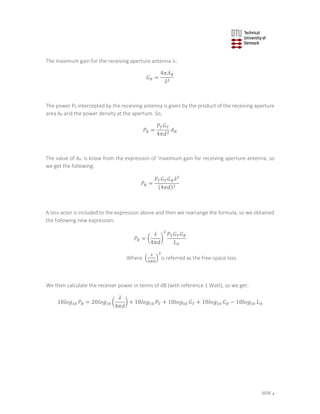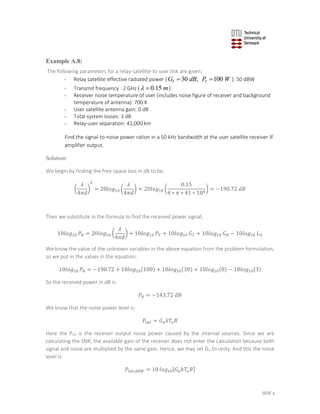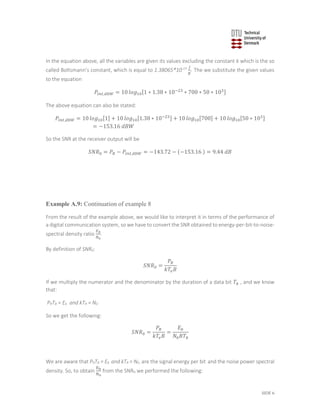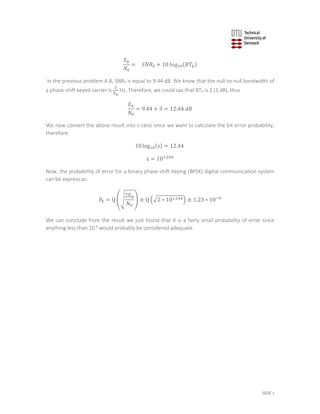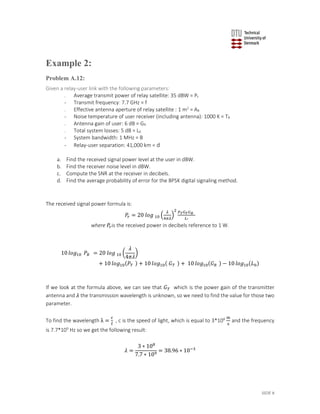Anzeige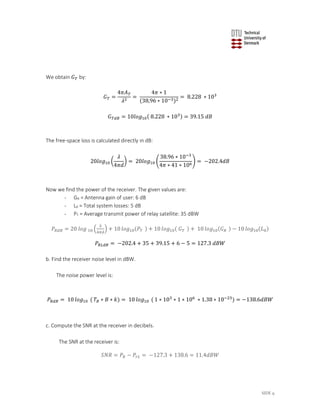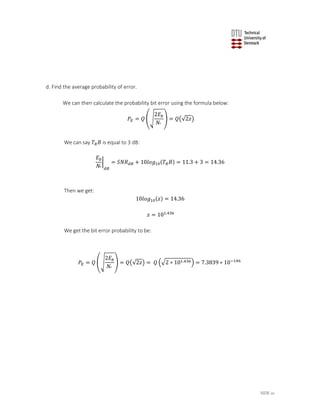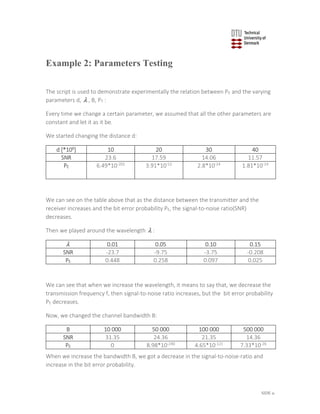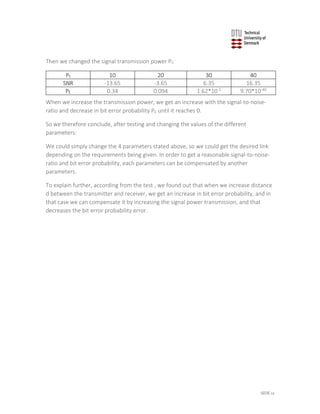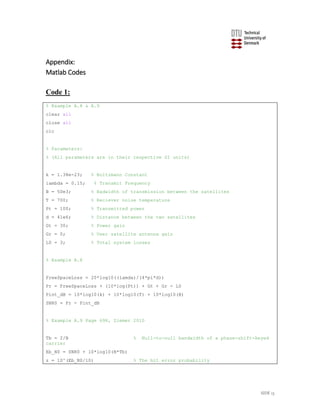Anzeige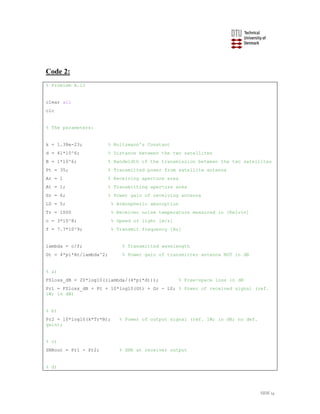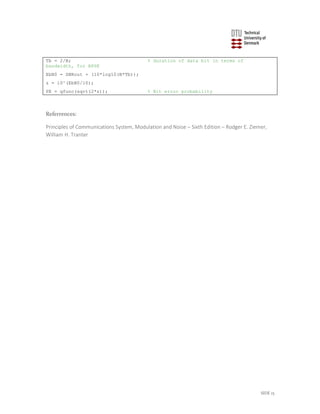Nächste SlideShare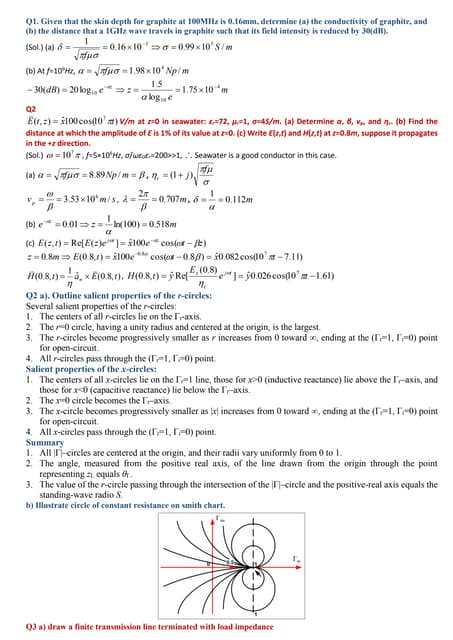Antenna Paper Solution
1 von 16
Anzeige

### Project 3 “Satellite Link Budgets and PE”

1. Arlene Meidahl - s107106 and Danish Bangash-s104712| Digital Communication | 21. maj 2015 Supervisor: John Aasted Sørensen Digital Communication and Modulation Project 3 “Satellite Link Budgets and PE”
2. SIDE 1 Satellite Link Budgets and PE The objective of this project is to construct a Matlab script for link budget determination for a satellite relay communication link, as shown in [Ziemer, 2010] Figure A.7 page 695: The script should include the following primary parameters for a satellite-satellite link: d Distance between the two satellites.  Transmitted wavelength. B Bandwidth of the transmission between the two satellites. TP Transmitted power from satellite antenna. TG Power gain of transmitter antenna over the isotropic radiation level. RA Receiving aperture area. RG Power gain of receiving antenna. 0L Atmospheric absorption. RT Receiver noise temperature.
3. SIDE 2 The script should allow for the determination of the SNR at the receiver output and the probability of bit error EP for the satellite link. The script is used to demonstrate experimentally the relation between EP and varying d ,  , B , TP by appropriately selected examples. It is suggested to use the Examples A.8 and A.9 at pages 697, 698 [Ziemer, 2010] as a starting point for examples. It is suggested to augment with at least two new examples created by yourself. The script should allow the user to determine the received power in dBW.
4. SIDE 3 Problem formulation: Considering a free-space electromagnetic-wave propagation channel as an example. For a better understanding how it works, we set our attention on the link between the two satellites which is the synchronous-orbit relay satellite and a low-orbit satellite or an aircraft as we can see in figure below. We assumed a relay satellite transmitted signal power of PT W. If we say that it is radiated isotropically, the power density at a distance d from the satellite is given by: 𝑃𝑡 = 𝑃𝑇 4𝜋𝑑2 [ 𝑊 𝑚2] If the radiated power is directed towards the low-orbit satellite or an aircraft as an example, then the maximum gain for the transmitting aperture antenna is: 𝐺 𝑇 = 4𝜋𝐴 𝑇 𝜆2
5. SIDE 4 The maximum gain for the receiving aperture antenna is: 𝐺 𝑅 = 4𝜋𝐴 𝑅 𝜆2 The power PR intercepted by the receiving antenna is given by the product of the receiving aperture area AR and the power density at the aperture. So, 𝑃𝑅 = 𝑃𝑇 𝐺 𝑇 4𝜋𝑑2 𝐴 𝑅 The value of AR is know from the expression of ‘maximum gain for receiving aperture antenna, so we get the following: 𝑃𝑅 = 𝑃𝑇 𝐺 𝑇 𝐺 𝑅 𝜆2 (4𝜋𝑑)2 A loss actor is included to the expression above and then we rearrange the formula, so we obtained the following new expression: 𝑃𝑅 = ( 𝜆 4𝜋𝑑 ) 2 𝑃𝑇 𝐺 𝑇 𝐺 𝑅 𝐿 𝑂 Where ( 𝜆 4𝜋𝑑 ) 2 is referred as the free-space loss. We then calculate the receiver power in terms of dB (with reference 1 Watt), so we get: 10𝑙𝑜𝑔10 𝑃𝑅 = 20𝑙𝑜𝑔10 ( 𝜆 4𝜋𝑑 ) + 10𝑙𝑜𝑔10 𝑃𝑇 + 10𝑙𝑜𝑔10 𝐺 𝑇 + 10𝑙𝑜𝑔10 𝐺 𝑅 − 10𝑙𝑜𝑔10 𝐿 𝑂
6. SIDE 5 Example A.8: The following parameters for a relay-satellite-to user link are given: - Relay satellite effective radiated power ( 30 ; 100T TG dB P W  ): 50 dBW - Transmit frequency : 2 GHz ( 0.15 m  ) - Receiver noise temperature of user (includes noise figure of receiver and background temperature of antenna): 700 K - User satellite antenna gain: 0 dB - Total system losses: 3 dB - Relay-user separation: 41,000 km Find the signal-to-noise power ration in a 50 kHz bandwidth at the user satellite receiver IF amplifier output. Solution: We begin by finding the free-space loss in dB to be: ( 𝜆 4𝜋𝑑 ) 2 = 20𝑙𝑜𝑔10 ( 𝜆 4𝜋𝑑 ) = 20𝑙𝑜𝑔10 ( 0.15 4 ∗ 𝜋 ∗ 41 ∗ 106 ) = −190.72 𝑑𝐵 Then we substitute in the formula to find the received power signal; 10𝑙𝑜𝑔10 𝑃𝑅 = 20𝑙𝑜𝑔10 ( 𝜆 4𝜋𝑑 ) + 10𝑙𝑜𝑔10 𝑃𝑇 + 10𝑙𝑜𝑔10 𝐺 𝑇 + 10𝑙𝑜𝑔10 𝐺 𝑅 − 10𝑙𝑜𝑔10 𝐿 𝑂 We know the value of the unknown variables in the above equation from the problem formulation, so we put in the values in the equation: 10𝑙𝑜𝑔10 𝑃𝑅 = −190.72 + 10𝑙𝑜𝑔10(100) + 10𝑙𝑜𝑔10(30) + 10𝑙𝑜𝑔10(0) − 10𝑙𝑜𝑔10(3) So the received power in dB is: 𝑃𝑅 = −143.72 𝑑𝐵 We know that the noise power level is: 𝑃𝑖𝑛𝑡 = 𝐺 𝑎 𝑘𝑇𝑒 𝐵 Here the Pint is the receiver output noise power caused by the internal sources. Since we are calculating the SNR, the available gain of the receiver does not enter the calculation because both signal and noise are multiplied by the same gain. Hence, we may set Ga to unity. And this the noise level is: 𝑃𝑖𝑛𝑡,𝑑𝑏𝑊 = 10 𝑙𝑜𝑔10[𝐺 𝑎 𝑘𝑇𝑒 𝐵]
7. SIDE 6 In the equation above, all the variables are given its values excluding the constant k which is the so called Boltsmann’s constant, which is equal to 1.38065*10-23 𝐽 𝐾 . The we substitute the given values to the equation 𝑃𝑖𝑛𝑡,𝑑𝑏𝑊 = 10 𝑙𝑜𝑔10[1 ∗ 1.38 ∗ 10−23 ∗ 700 ∗ 50 ∗ 103] The above equation can also be stated: 𝑃𝑖𝑛𝑡,𝑑𝑏𝑊 = 10 𝑙𝑜𝑔10 + 10 𝑙𝑜𝑔10[1.38 ∗ 10−23] + 10 𝑙𝑜𝑔10 + 10 𝑙𝑜𝑔10[50 ∗ 103] = −153.16 𝑑𝐵𝑊 So the SNR at the receiver output will be 𝑆𝑁𝑅0 = 𝑃𝑅 − 𝑃𝑖𝑛𝑡,𝑑𝑏𝑊 = −143.72 − (−153.16 ) = 9.44 𝑑𝐵 Example A.9: Continuation of example 8 From the result of the example above, we would like to interpret it in terms of the performance of a digital communication system, so we have to convert the SNR obtained to energy-per-bit-to-noise- spectral density ratio 𝐸 𝑏 𝑁0 . By definition of SNR0: 𝑆𝑁𝑅0 = 𝑃𝑅 𝑘𝑇𝑒 𝐵 If we multiply the numerator and the denominator by the duration of a data bit 𝑇𝑏 , and we know that: PRTB = Eb and kTR = N0 So we get the following: 𝑆𝑁𝑅0 = 𝑃𝑅 𝑘𝑇𝑒 𝐵 = 𝐸 𝑏 𝑁0 𝐵𝑇𝑏 We are aware that PRTB = Eb and kTR = N0 , are the signal energy per bit and the noise power spectral density. So, to obtain Eb N0 from the SNR0 we performed the following:
8. SIDE 7 𝐸 𝑏 𝑁0 = 𝑆𝑁𝑅0 + 10 𝑙𝑜𝑔10(𝐵𝑇𝑏) In the previous problem A.8, SNR0 is equal to 9.44 dB. We know that the null-to-null bandwidth of a phase-shift keyed carrier is 2 Tb Hz. Therefore, we could say that BTb is 2 (3 dB), thus 𝐸 𝑏 𝑁0 = 9.44 + 3 = 12.44 𝑑𝐵 We now convert the above result into z-ratio since we want to calculate the bit error probability, therefore 10 log10(z) = 12.44 z = 101.244 Now, the probability of error for a binary phase-shift keying (BPSK) digital communication system can be express as: PE = Q (√ 2Eb N0 ) ≅ Q (√2 ∗ 101.244) ≅ 1.23 ∗ 10−9 We can conclude from the result we just found that it is a fairly small probability of error since anything less than 10-6 would probably be considered adequate.
9. SIDE 8 Example 2: Problem A.12: Given a relay-user link with the following parameters: - Average transmit power of relay satellite: 35 dBW = Pt - Transmit frequency: 7.7 GHz = f - Effective antenna aperture of relay satellite : 1 m2 = AR - Noise temperature of user receiver (including antenna): 1000 K = TR - Antenna gain of user: 6 dB = GR - Total system losses: 5 dB = L0 - System bandwidth: 1 MHz = B - Relay-user separation: 41,000 km = d a. Find the received signal power level at the user in dBW. b. Find the receiver noise level in dBW. c. Compute the SNR at the receiver in decibels. d. Find the average probability of error for the BPSK digital signaling method. The received signal power formula is: 𝑃𝑟 = 20 𝑙𝑜𝑔 10 ( 𝜆 4𝜋𝜆 ) 2 𝑃 𝑇 𝐺 𝑇 𝐺 𝑅 𝐿° where 𝑃𝑟is the received power in decibels reference to 1 W. 10 𝑙𝑜𝑔10 𝑃𝑅 = 20 𝑙𝑜𝑔 10 ( 𝜆 4𝜋𝜆 ) + 10 𝑙𝑜𝑔10(𝑃𝑇 ) + 10 𝑙𝑜𝑔10( 𝐺 𝑇 ) + 10 𝑙𝑜𝑔10(𝐺 𝑅 ) − 10 𝑙𝑜𝑔10(𝐿0) If we look at the formula above, we can see that 𝐺 𝑇 which is the power gain of the transmitter antenna and 𝜆 the transmission wavelength is unknown, so we need to find the value for those two parameter. To find the wavelength λ = c f , c is the speed of light, which is equal to 3*108 m s and the frequency is 7.7*109 Hz so we get the following result: 𝜆 = 3 ∗ 108 7.7 ∗ 109 = 38.96 ∗ 10−3
10. SIDE 9 We obtain 𝐺 𝑇 by: 𝐺 𝑇 = 4𝜋𝐴 𝑇 𝜆2 = 4𝜋 ∗ 1 (38,96 ∗ 10−3)2 = 8.228 ∗ 103 𝐺 𝑇𝑑𝐵 = 10𝑙𝑜𝑔10( 8.228 ∗ 103) = 39.15 𝑑𝐵 The free-space loss is calculated directly in dB: 20𝑙𝑜𝑔10 ( 𝜆 4𝜋𝑑 ) = 20𝑙𝑜𝑔10 ( 38.96 ∗ 10−3 4𝜋 ∗ 41 ∗ 106) = −202.4𝑑𝐵 Now we find the power of the receiver. The given values are: - GR = Antenna gain of user: 6 dB - L0 = Total system losses: 5 dB - PT = Average transmit power of relay satellite: 35 dBW 𝑃𝑅𝑑𝐵 = 20 𝑙𝑜𝑔 10 ( 𝜆 4𝜋𝜆 ) + 10 𝑙𝑜𝑔10(𝑃𝑇 ) + 10 𝑙𝑜𝑔10( 𝐺 𝑇 ) + 10 𝑙𝑜𝑔10(𝐺 𝑅 ) − 10 𝑙𝑜𝑔10(𝐿0) 𝑃𝑅𝐿𝑑𝐵 = −202.4 + 35 + 39.15 + 6 − 5 = 127.3 𝑑𝐵𝑊 b. Find the receiver noise level in dBW. The noise power level is: 𝑃𝑅𝑑𝐵 = 10 𝑙𝑜𝑔10 ( 𝑇𝑅 ∗ 𝐵 ∗ 𝑘) = 10 𝑙𝑜𝑔10 ( 1 ∗ 103 ∗ 1 ∗ 106 ∗ 1.38 ∗ 10−23 ) = −138.6𝑑𝐵𝑊 c. Compute the SNR at the receiver in decibels. The SNR at the receiver is: 𝑆𝑁𝑅 = 𝑃𝑅 − 𝑃𝑟𝐿 = −127.3 + 138.6 = 11.4𝑑𝐵𝑊
11. SIDE 10 d. Find the average probability of error. We can then calculate the probability bit error using the formula below: 𝑃𝐸 = 𝑄 (√ 2𝐸 𝑏 𝑁° ) = 𝑄(√2𝑧) We can say 𝑇𝑅 𝐵 is equal to 3 dB: 𝐸 𝑏 𝑁° | 𝑑𝐵 = 𝑆𝑁𝑅 𝑑𝐵 + 10𝑙𝑜𝑔10(𝑇𝑅 𝐵) = 11.3 + 3 = 14.36 Then we get: 10𝑙𝑜𝑔10(𝑧) = 14.36 𝑧 = 101.436 We get the bit error probability to be: 𝑃𝐸 = 𝑄 (√ 2𝐸 𝑏 𝑁° ) = 𝑄(√2𝑧) = 𝑄 (√2 ∗ 101.436) = 7.3839 ∗ 10−146
12. SIDE 11 Example 2: Parameters Testing The script is used to demonstrate experimentally the relation between PE and the varying parameters d,  , B, PT : Every time we change a certain parameter, we assumed that all the other parameters are constant and let it as it be. We started changing the distance d: d [*106] 10 20 30 40 SNR 23.6 17.59 14.06 11.57 PE 6.49*10-202 3.91*10-52 2.8*10-24 1.81*10-14 We can see on the table above that as the distance between the transmitter and the receiver increases and the bit error probability PE, the signal-to-noise ratio(SNR) decreases. Then we played around the wavelength  :  0.01 0.05 0.10 0.15 SNR -23.7 -9.75 -3.75 -0.208 PE 0.448 0.258 0.097 0.025 We can see that when we increase the wavelength, it means to say that, we decrease the transmission frequency f, then signal-to-noise ratio increases, but the bit error probability PE decreases. Now, we changed the channel bandwidth B: B 10 000 50 000 100 000 500 000 SNR 31.35 24.36 21.35 14.36 PE 0 8.98*10-240 4.65*10-121 7.33*10-26 When we increase the bandwidth B, we got a decrease in the signal-to-noise-ratio and increase in the bit error probability.
13. SIDE 12 Then we changed the signal transmission power Pt: Pt 10 20 30 40 SNR -13.65 -3.65 6.35 16.35 PE 0.34 0.094 1.62*10-5 9.70*10-40 When we increase the transmission power, we get an increase with the signal-to-noise- ratio and decrease in bit error probability PE until it reaches 0. So we therefore conclude, after testing and changing the values of the different parameters: We could simply change the 4 parameters stated above, so we could get the desired link depending on the requirements being given. In order to get a reasonable signal-to-noise- ratio and bit error probability, each parameters can be compensated by another parameters. To explain further, according from the test , we found out that when we increase distance d between the transmitter and receiver, we get an increase in bit error probability, and in that case we can compensate it by increasing the signal power transmission, and that decreases the bit error probability error.
14. SIDE 13 Appendix: Matlab Codes Code 1: % Example A.8 & A.9 clear all close all clc % Parameters: % (All parameters are in their respective SI units) k = 1.38e-23; % Boltzmann Constant lambda = 0.15; % Transmit Frequency B = 50e3; % Badwidth of transmission between the satellites T = 700; % Reciever noise temperature Pt = 100; % Transmitted power d = 41e6; % Distance between the two satellites Gt = 30; % Power gain Gr = 0; % User satellite antenna gain L0 = 3; % Total system losses % Example A.8 FreeSpaceLoss = 20*log10((lamda)/(4*pi*d)) Pr = FreeSpaceLoss + (10*log(Pt)) + Gt + Gr - L0 Pint_dB = 10*log10(k) + 10*log10(T) + 10*log10(B) SNR0 = Pr - Pint_dB % Example A.9 Page 698, Ziemer 2010 Tb = 2/B % Null-to-null bandwidth of a phase-shift-keyed carrier Eb_N0 = SNR0 + 10*log10(B*Tb) z = 10^(Eb_N0/10) % The bit error probability
15. SIDE 14 Code 2: % Problem A.12 clear all clc % The parameters: k = 1.38e-23; % Boltzmann's Constant d = 41*10^6; % Distance between the two satellites B = 1*10^6; % Bandwidth of the transmission between the two satellites Pt = 35; % Transmitted power from satellite antenna Ar = 1 % Receiving aperture area At = 1; % Transmitting aperture area Gr = 6; % Power gain of receiving antenna L0 = 5; % Atmospheric absorption Tr = 1000 % Receiver noise temperature measured in [Kelvin] c = 3*10^8; % Speed of light [m/s] f = 7.7*10^9; % Transmit frequency [Hz] lambda = c/f; % Transmitted wavelength Gt = 4*pi*At/lambda^2; % Power gain of transmitter antenna NOT in dB % a) FSloss_dB = 20*log10((lambda/(4*pi*d))); % Free-space loss in dB Pr1 = FSloss_dB + Pt + 10*log10(Gt) + Gr - L0; % Power of received signal (ref. 1W; in dB) % b) Pr2 = 10*log10(k*Tr*B); % Power of output signal (ref. 1W; in dB; no def. gain); % c) SNRout = Pr1 - Pr2; % SNR at receiver output % d)
16. SIDE 15 Tb = 2/B; % duration of data bit in terms of bandwidth, for BPSK EbN0 = SNRout + (10*log10(B*Tb)); z = 10^(EbN0/10); PE = qfunc(sqrt(2*z)); % Bit error probability Referrences: Principles of Communications System, Modulation and Noise – Sixth Edition – Rodger E. Ziemer, William H. Tranter
Anzeige